# Coursera: Machine Learning (Week 3) [Assignment Solution] - Andrew NG

▸ Logistic regression and apply it to two different datasets.

I have recently completed the Machine Learning course from Coursera by Andrew NG.

While doing the course we have to go through various quiz and assignments.

Here, I am sharing my solutions for the weekly assignments throughout the course.

These solutions are for reference only.

It is recommended that you should solve the assignments by yourself honestly then only it makes sense to complete the course.
But, In case you stuck in between, feel free to refer the solutions provided by me.

#### NOTE:

Don't just copy paste the code for the sake of completion.
Even if you copy the code, make sure you understand the code first.

Click here to check out week-2 assignment solutions, Scroll down for the solutions for week-3 assignment.

In this exercise, you will implement logistic regression and apply it to two different datasets. Before starting on the programming exercise, we strongly recommend watching the video lectures and completing the review questions for the associated topics.

It consist of the following files:
• ex2.m - Octave/MATLAB script that steps you through the exercise
• ex2 reg.m - Octave/MATLAB script for the later parts of the exercise
• ex2data1.txt - Training set for the first half of the exercise
• ex2data2.txt - Training set for the second half of the exercise
• submit.m - Submission script that sends your solutions to our servers
• mapFeature.m - Function to generate polynomial features
• plotDecisionBoundary.m - Function to plot classifier's decision boundary
• Function to plot 2D classification data
• Sigmoid Function
• Logistic Regression Cost Function
• Logistic Regression Prediction Function
• Regularized Logistic Regression Cost
• YouTube videos featuring Free IOT/ML tutorials
* indicates files you will need to complete

### plotData.m :

```function plotData(X, y)
%PLOTDATA Plots the data points X and y into a new figure
%   PLOTDATA(x,y) plots the data points with + for the positive examples
%   and o for the negative examples. X is assumed to be a Mx2 matrix.

% ====================== YOUR CODE HERE ======================
% Instructions: Plot the positive and negative examples on a
%               2D plot, using the option 'k+' for the positive
%               examples and 'ko' for the negative examples.
%

%Seperating positive and negative results
pos = find(y==1); %index of positive results
neg = find(y==0); %index of negative results

% Create New Figure
figure;

%Plotting Positive Results on
%    X_axis: Exam1 Score =  X(pos,1)
%    Y_axis: Exam2 Score =  X(pos,2)
plot(X(pos,1),X(pos,2),'g+');

%To keep above plotted graph as it is.
hold on;

%Plotting Negative Results on
%    X_axis: Exam1 Score =  X(neg,1)
%    Y_axis: Exam2 Score =  X(neg,2)
plot(X(neg,1),X(neg,2),'ro');

% =========================================================================

hold off;
end```

### sigmoid.m :

```function g = sigmoid(z)
%SIGMOID Compute sigmoid function
%   g = SIGMOID(z) computes the sigmoid of z.

% You need to return the following variables correctly
g = zeros(size(z));

% ====================== YOUR CODE HERE ======================
% Instructions: Compute the sigmoid of each value of z (z can be a matrix,
%               vector or scalar).
g = 1./(1+exp(-z));

% =============================================================
end```

### costFunction.m :

```function [J, grad] = costFunction(theta, X, y)
%COSTFUNCTION Compute cost and gradient for logistic regression
%   J = COSTFUNCTION(theta, X, y) computes the cost of using theta as the
%   parameter for logistic regression and the gradient of the cost
%   w.r.t. to the parameters.

% Initialize some useful values
m = length(y); % number of training examples

% You need to return the following variables correctly
J = 0;

% ====================== YOUR CODE HERE ======================
% Instructions: Compute the cost of a particular choice of theta.
%               You should set J to the cost.
%               Compute the partial derivatives and set grad to the partial
%               derivatives of the cost w.r.t. each parameter in theta
%
% Note: grad should have the same dimensions as theta
%
%DIMENSIONS:
%   theta = (n+1) x 1
%   X     = m x (n+1)
%   y     = m x 1
%   grad  = (n+1) x 1
%   J     = Scalar

z = X * theta;      % m x 1
h_x = sigmoid(z);   % m x 1

J = (1/m)*sum((-y.*log(h_x))-((1-y).*log(1-h_x))); % scalar

grad = (1/m)* (X'*(h_x-y));     % (n+1) x 1

% =============================================================

end```

### predict.m :

```function p = predict(theta, X)
%PREDICT Predict whether the label is 0 or 1 using learned logistic
%regression parameters theta
%   p = PREDICT(theta, X) computes the predictions for X using a
%   threshold at 0.5 (i.e., if sigmoid(theta'*x) >= 0.5, predict 1)

m = size(X, 1); % Number of training examples

% You need to return the following variables correctly
p = zeros(m, 1);

% ====================== YOUR CODE HERE ======================
% Instructions: Complete the following code to make predictions using
%               your learned logistic regression parameters.
%               You should set p to a vector of 0's and 1's
%
% Dimentions:
% X     =  m x (n+1)
% theta = (n+1) x 1

h_x = sigmoid(X*theta);
p=(h_x>=0.5);

%p = double(sigmoid(X * theta)>=0.5);
% =========================================================================
end```

### costFunctionReg.m :

```function [J, grad] = costFunctionReg(theta, X, y, lambda)
%COSTFUNCTIONREG Compute cost and gradient for logistic regression with regularization
%   J = COSTFUNCTIONREG(theta, X, y, lambda) computes the cost of using
%   theta as the parameter for regularized logistic regression and the
%   gradient of the cost w.r.t. to the parameters.

% Initialize some useful values
m = length(y); % number of training examples

% You need to return the following variables correctly
J = 0;

% ====================== YOUR CODE HERE ======================
% Instructions: Compute the cost of a particular choice of theta.
%               You should set J to the cost.
%               Compute the partial derivatives and set grad to the partial
%               derivatives of the cost w.r.t. each parameter in theta

%DIMENSIONS:
%   theta = (n+1) x 1
%   X     = m x (n+1)
%   y     = m x 1
%   grad  = (n+1) x 1
%   J     = Scalar

z = X * theta;      % m x 1
h_x = sigmoid(z);  % m x 1

reg_term = (lambda/(2*m)) * sum(theta(2:end).^2);

J = (1/m)*sum((-y.*log(h_x))-((1-y).*log(1-h_x))) + reg_term; % scalar

grad(1) = (1/m)* (X(:,1)'*(h_x-y));                                  % 1 x 1
grad(2:end) = (1/m)* (X(:,2:end)'*(h_x-y))+(lambda/m)*theta(2:end);  % n x 1

% =============================================================
end```

I tried to provide optimized solutions like vectorized implementation for each assignment. If you think that more optimization can be done, then put suggest the corrections / improvements.

--------------------------------------------------------------------------------
&
Click here to see more codes for Raspberry Pi 3 and similar Family.
&
Click here to see more codes for NodeMCU ESP8266 and similar Family.
&
Click here to see more codes for Arduino Mega (ATMega 2560) and similar Family.

Feel free to ask doubts in the comment section. I will try my best to solve it.
If you find this helpful by any mean like, comment and share the post.
This is the simplest way to encourage me to keep doing such work.

Thanks and Regards,
-Akshay P. Daga

1.how could you do this please explain me...

1.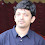What explanation you want?

2.1.You can copy the the code from above code sections.

3.Hi Akshay, Please may I have theses files as well:

ex2.m
ex2 reg.m
ex2data1.txt
ex2data2.txt
submit.m
mapFeature.m
plotDecisionBoundary.m

1.You can get those files from Coursera assignments. I don't have those with me now.

4.can you please tell me what you did by this

1.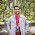its the simplified version of derivative term d/d0*j0 which we call gradient. check the formula once and you will understand it

5.this means:- take the transpose of feature matrix X(i.e X') and multiply it with the difference of matrices h_x and y i.e the matrix with sigmoid outputs and the result matrix(y). Finally multiply the end product with 1/m , where m is the number of training examples.

This is the vectorized implementation of the code that's actually way more lengthier to implement using loops.

6.Hi, can you please explain the predict function?

7.In this gradient decent the number of iteration are not specified so how is the gradient decent working? can someone please explain?

8.I used the exact code at the end but I'm still getting 65/100 not able to figure out the reason

1.Did you figure out the reason yet?

9.Hi !! why didn't you use sum() function for grad even why formula contains that ?

1.sum() is used for the summation in the formula.
Here We are doing matrix multiplication which itself consist of "sum of product". So, no need of external sum function.
Please try to do it on paper by yourself, you will get clear idea.
Thanks

10.we have learned that Z= theta transpose X then why are using Z=X multiplied by theta in the above codes ?

1.When we are calculating z(small z) for a single sample, then it is z=theta' * x. (here small x)
But When you do the same computation for all the samples at the same time then we call it as Z (Capital Z).
Z = X * theta. (Here Capital X)

Try to do it using pen-paper, you will get clear understanding.

11.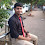I tried coding for predict function in the following way:

h_x = sigmoid(X*theta);
if (0<=h_x<0.5)
p=0;
elseif (0.5<=h_x<=1)
p=1;
endif

I know I did it in a long way but the accuracy that I am getting 60.00. Your code gave me the accuracy 89.00. Can you please help me understand what's wrong with this and what's the exact difference between your code and mines'?

1.P is a matrix with dimensions m x 1.
Solution:
You can put your code in a "for" loop and check the value of each element in h_x and accordingly set the value of each element in p.

It will work.

12.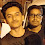hey bro it says z not defined why???

1.Hi, I think you are doing this assignment in Octave and that's why you are facing this issue.

Chethan Bhandarkar has provided solution for it. Please check it out: https://www.apdaga.com/2018/06/coursera-machine-learning-week-2.html?showComment=1563986935868#c4682866656714070064

Thanks

13.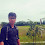I have copy the exact code for plotData.m , and all the others program worked very well but I am still getting 70/100. Can you tel what's the problem ?

14.Can you tell me , how can I run "ex2" script in console ?

15.hi I want to clarify few things from you,
I have read in regression, these are few important points which have not been covered in andrew ng regression topic, how to find how significant your variable is, significance of p value and R^2 (R-square) values. I would like to know more about them. kindly share some sources.

16.HI, The line code reg_term = (lambda/(2*m)) * sum(theta(2:end).^2); in costFunctionReg function,

can you explain more about this part theta(2:end) , what does it mean and how did you deduce it,

17.I used
for i=1:size(X,1)
if sigmoid(X*theta)>=0.5
p=sigmoid(X*theta);

end
as well as,
h_x = sigmoid(X*theta);
for i=1:size(X,1)

if (0<=h_x<0.5)
p=0;
elseif (0.5<=h_x<=1)
p=1;
end
but i am getting 40 accuracy it is working only with your code.why sir?

18.Hi there,
I am trying the the same code as yours of sigmoid() function but each time it is getting an error saying that

'z' undefined near line 6 column 18
error: called from
sigmoid at line 6 column 5

19.Hello Akshay,
It'd be great if you kindly share the code for "fminunc" in this week's files(wherever needed), coz i don't understand that particular function well, neither did i get its solution anywhere else on internet.

1.Hi Ankit,
Sorry but I don't have the code for "fminunc".

20.21.Hey it says my plot is empty can someone help?

22.I am facing this type of problem in matlab , what can i do ? how to fix that n where ??

'fminunc' requires Optimization Toolbox.

Error in ex2 (line 99)
fminunc(@(t)(costFunction(t, X, y)), initial_theta, options);

23.In sigmoid
error in line 6 (the preallocated value assigned to variable 'g' might be unused)

what should i do

1.How's value of 'g' is unused. 'g' is nothing but output of sigmoid function.
If you are getting some msg, it must be warning not error. So, don't worry about it, keep it as it is. (But I don't think you should get any kind of warning like this).
line 6, is called initialization of variable.

24.Hi Akshay can you please explain why we use this X(:,2:end) and theta(2:end) instead of plain X and theta??

1.It's because as per the theory in videos, We don't apply regularization on theta_0. Regularization is applied from theta_1 onwards.
and that's why 2 gradients. 1st corresponding to theta_0 and other for theta_1 onwards.

25.And also why use two gradents?

26.Good day sir,
im new in this course...i could not fully understand the assignment in week 3...as i enter my code...i think still in error..

27.1.Predict function is fairly simple. You have implemented your gradient and now you just have to predict whether the answer will be 1 or 0... So, what will you do is check for the result > 0.5. If it is above the 0.5, then prediction will be true (1), otherwise false (0)

2.@Hassan Ashas Thank you very much for your explanation.

28.costfuntion is not returning the scalar value, it is returning the 1*100 matrix.

29.Hello Akshay,
I keep getting this error for the costFunctionReg.m file:

syntax error
>>> reg_term = (lambda/2*m)) * sum(theta(2:end).^2);
^
What is the problem here I do not understand.

Thank you

1.Opening and closing brackets are not matching you code.

NOTE: check the brackets are "2*m"

YOUR CODE: reg_term = (lambda/2*m)) * sum(theta(2:end).^2);
WORKING CODE: reg_term = (lambda/(2*m)) * sum(theta(2:end).^2);

30.Hello Akshay,
While computing cost function I am getting so many outputs

1.You should only get [J, grad] as a output of costFunction & costFunctionReg.

31.Error - theta may not be defined , predict function

32.hi i have a doubt i took theta as [zeros(n+1),1] it is giving me 0 and i cant submit the assignment can you specify initial value of theta and theta and values of X. i am totally confused

33.nothing is working here
every time it is showing
>> plotData

error: 'y' undefined near line 14 column 12
error: called from
plotData at line 14 column 5
>>

34.J = (1 / m) * sum ((- y. * Log (h_x)) - ((1-y). * Log (1-h_x))) the log representation in this equation means ln isn't it? So, shouldn't we write it as log (1-h_x) / log (10).

35.function [J, grad] = costFunctionReg(theta, X, y, lambda)
%COSTFUNCTIONREG Compute cost and gradient for logistic regression with regularization
% J = COSTFUNCTIONREG(theta, X, y, lambda) computes the cost of using
% theta as the parameter for regularized logistic regression and the
% gradient of the cost w.r.t. to the parameters.

% Initialize some useful values
m = length(y); % number of training examples

% You need to return the following variables correctly
J = 0;

% ====================== YOUR CODE HERE ======================
% Instructions: Compute the cost of a particular choice of theta.
% You should set J to the cost.
% Compute the partial derivatives and set grad to the partial
% derivatives of the cost w.r.t. each parameter in theta

[J, grad] = costFunction(theta, X, y);
feats = theta(2:end);
J = J + lambda / (2 * m) * (feats' * feats);

% =============================================================

end

36.My question is about the solved subroutine 'plotDecisionBoundary.m'
Line 20 : plot_y
I didn't understand the definition of this
Infact how this particular code helped to plot the decision boundary! Please explain..

37.so in cost function grad is basically you doing gradient descent right? but what is the use of 1/m? i'm really confused sorry

1.While calculating cost function, we are doing sum (summation) operation over 'm' samples. And then dividing it by 'm' in order to scale the output (as a scaling factor).

38.Muje 55 marks hi aa rahe he mane code bhi sahi likha he phir bhi...logistic regression cost and regularised logistic regression gradient dono me 0 marks he..

39.i really confused in assignment, i enjoyed all the stuff that prof.Ng doing buat why it turns out to become nightmare when im face the programming assignment?
In the cosfunctionreg.m why you put

grad(1) = (1/m)* (X(:,1)'*(h_x-y)); whats this mean?

1.These 2 lines are for calcuating gradient with regularization.
since we don't add regularization term to 1st entry. (we have to write 2 seperate lines of code for it)

40.Hi dear Akshay. I'm trying to submit week 3 assignment but I keep seeing the error:
!! Submission failed: unexpected error: Error: File: costFunctionReg.m Line: 22 Column: 3
Invalid expression. Check for missing or extra characters.
Can you help me out?

41.I am getting a syntax error in exercise "CostfunctionReg.m" at
please tell me why am i getting this error.
yes i am running it in octave but please don't tell me to go through the another link . please just tell me the issue.

42.!! Submission failed: Index exceeds array bounds.

Function: getResponse

LineNumber: 132of submitWithConfiguration

43.Here in the cost function though y and log(h_x) both have the same dimensions (mx1), how the dot product is possible between them?

1.We are not doing the dot product of y and log(h_x) while calculating cost function. Multiplication represented by dot astrix (.*) means element wise multiplication in matlab.
Eg. -y.*log(h_x)
Please check the code once again.